﻿ 船舶单桨工况拖转调距桨性能计算及其对快速性的影响分析
 舰船科学技术2018, Vol. 40Issue (4): 48-52,57PDF

Simulation on the steady-state and dynamic characteristics of single propeller working condition of ship propulsion system
XIONG Kai-jun
The Navy in Jiujiang Region Military Agent's Room, Jiujiang 332007, China
Abstract: The open water performance of controllable pitch propeller (CPP) is numerically simulated by CFD method, and the result is verified by the test data. When the advance ratio is big the open water performance is attained by both CFD method and conic approach arithmetic, which extending the range of the open water performance curve. The mathematic models of main engine, transmission system, controllable pitch propeller and hull resistance are developed, and the " hull-propeller-engine” propulsion system simulation model is integrated in Simulink. The steady-state characteristics of single propeller working condition is simulated, in which the resistance of the unworking propeller is studied chiefly, the simulation results show that: When the unworking propeller is free running, the resistance added by the unworking propeller is the smallest and the speed-ability is the best when the pitch of this propeller is at the maximal position. The ship speed and the pitch of the unworking propeller are the two major factors which influence the performances of the unworking propeller. With the increasing of ship speed, the added dragged resistance, torque and rev of the unworking propeller increase too, and the increasing of the pitch of the unworking propeller will cause the reduced free running propeller’s dragged resistance, the torque and rev increase appreciably at first and then decrease to the minimum value.
Key words: ship propulsion system     single propeller working condition     CPP     simulation
0 引　言

1 调距桨水动力性能数值计算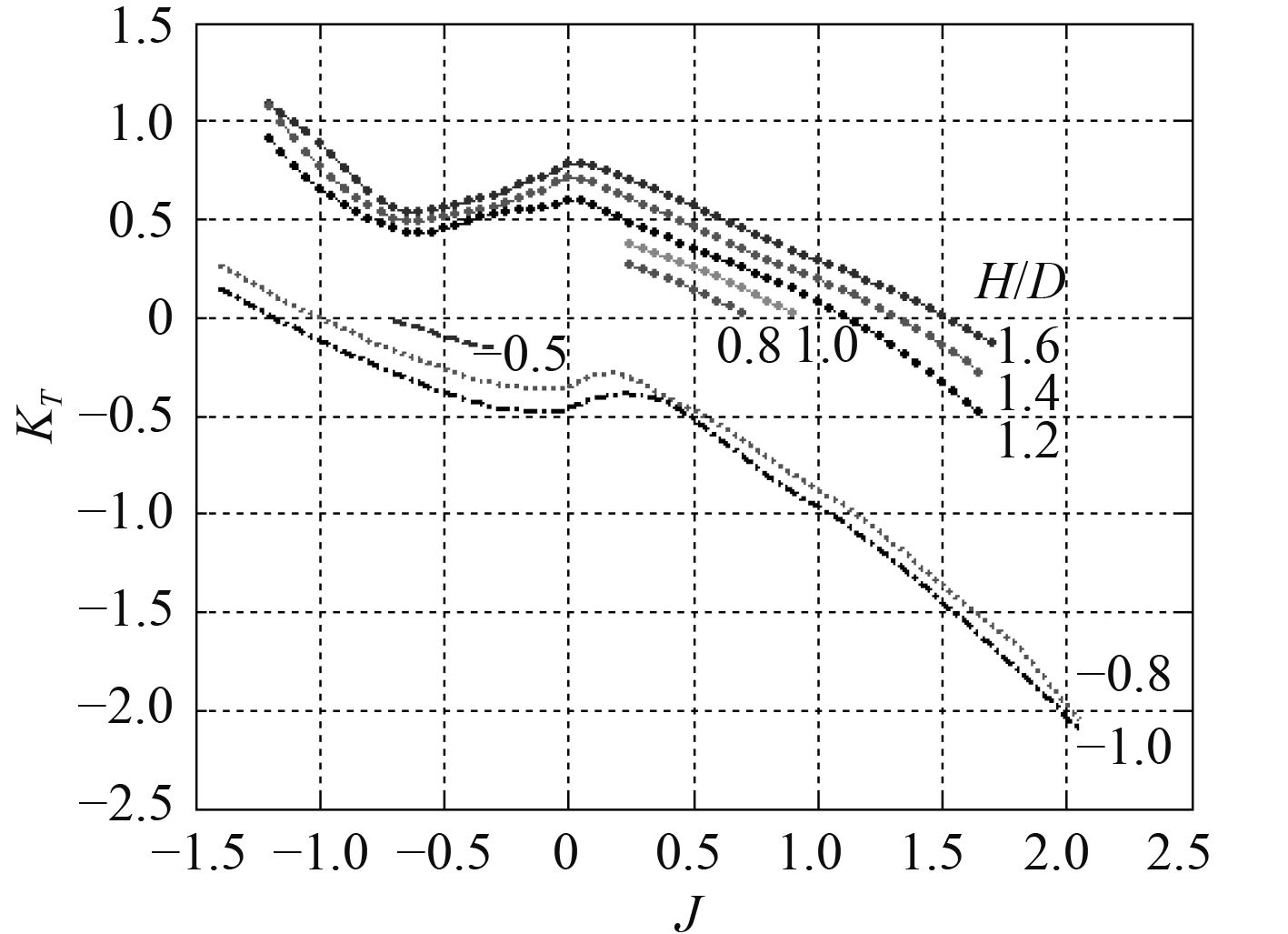图 1 某调距桨桨敞水特性试验曲线 Fig. 1 Open water performance of CPP from test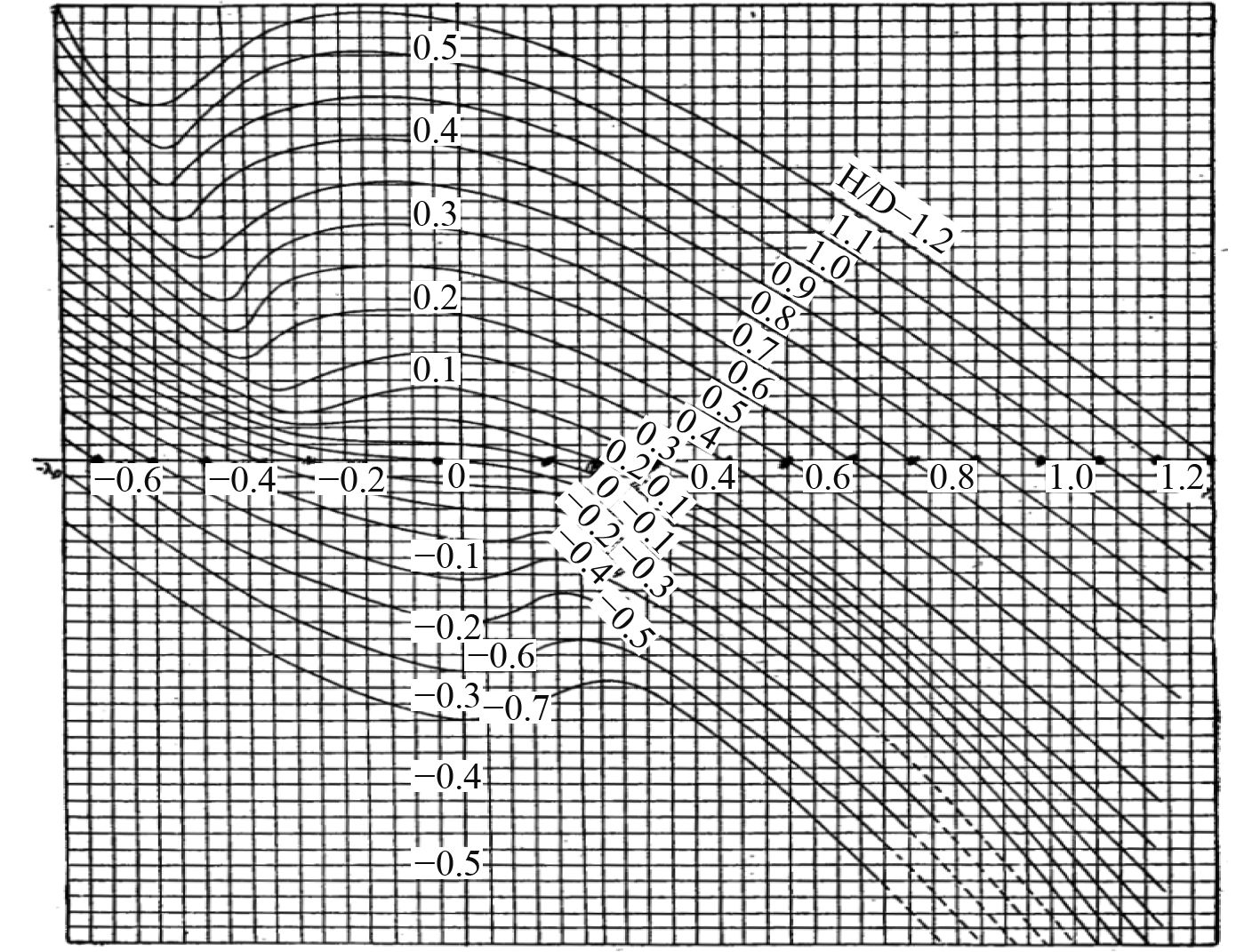图 2 完整的调距桨敞水特性曲线 Fig. 2 Whole open water performance of CPP

 $\frac{{\partial {u_i}}}{{\partial {x_i}}} = 0\text{，}$ (1)
 $\begin{split}& \frac{\partial }{{\partial t}}(\rho {u_i}) + \frac{\partial }{{\partial {x_j}}}(\rho {u_j}{u_i}) = - \frac{{\partial p}}{{\partial {x_i}}} + \\& \frac{\partial }{{\partial {x_j}}}[(\mu + {\mu _t})(\frac{{\partial {u_i}}}{{\partial {x_j}}} + \frac{{\partial {u_j}}}{{\partial {x_i}}})] + {f_i}\text{。}\end{split}$ (2)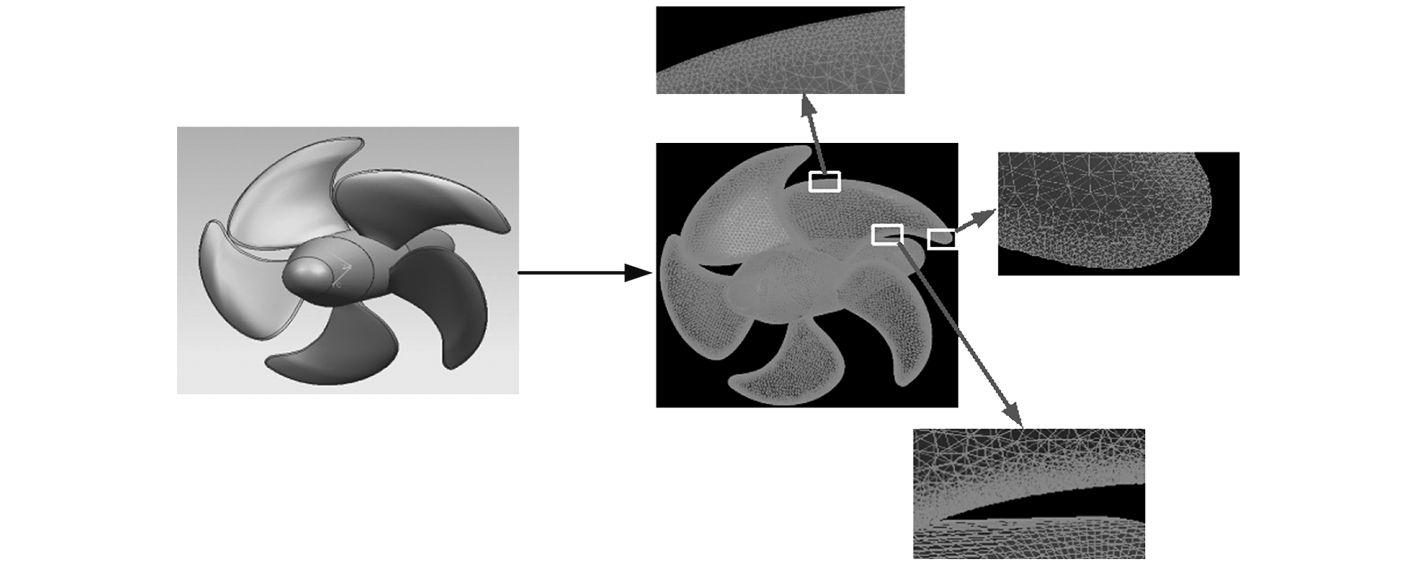图 3 调距桨几何建模与计算网格划分 Fig. 3 Geometrical model and calculating grid of CPP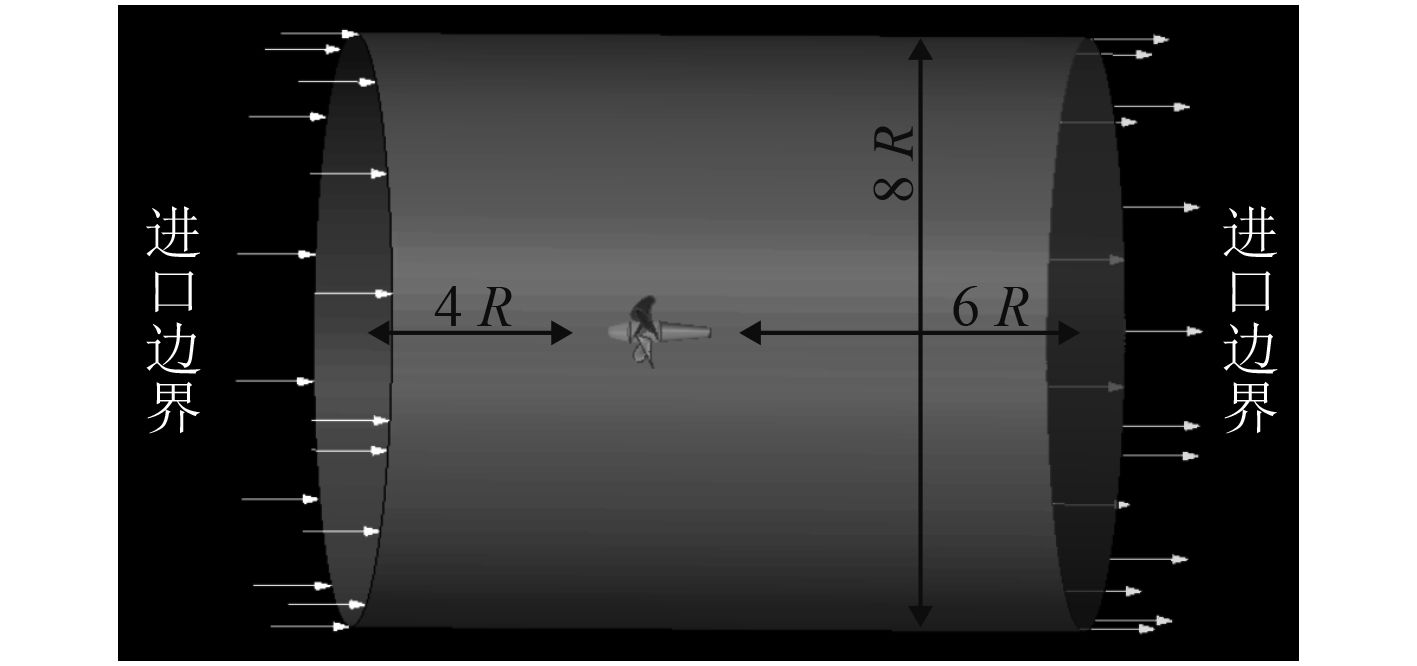图 4 计算区域与调距桨的相对位置 Fig. 4 Relative position of CPP and calculating region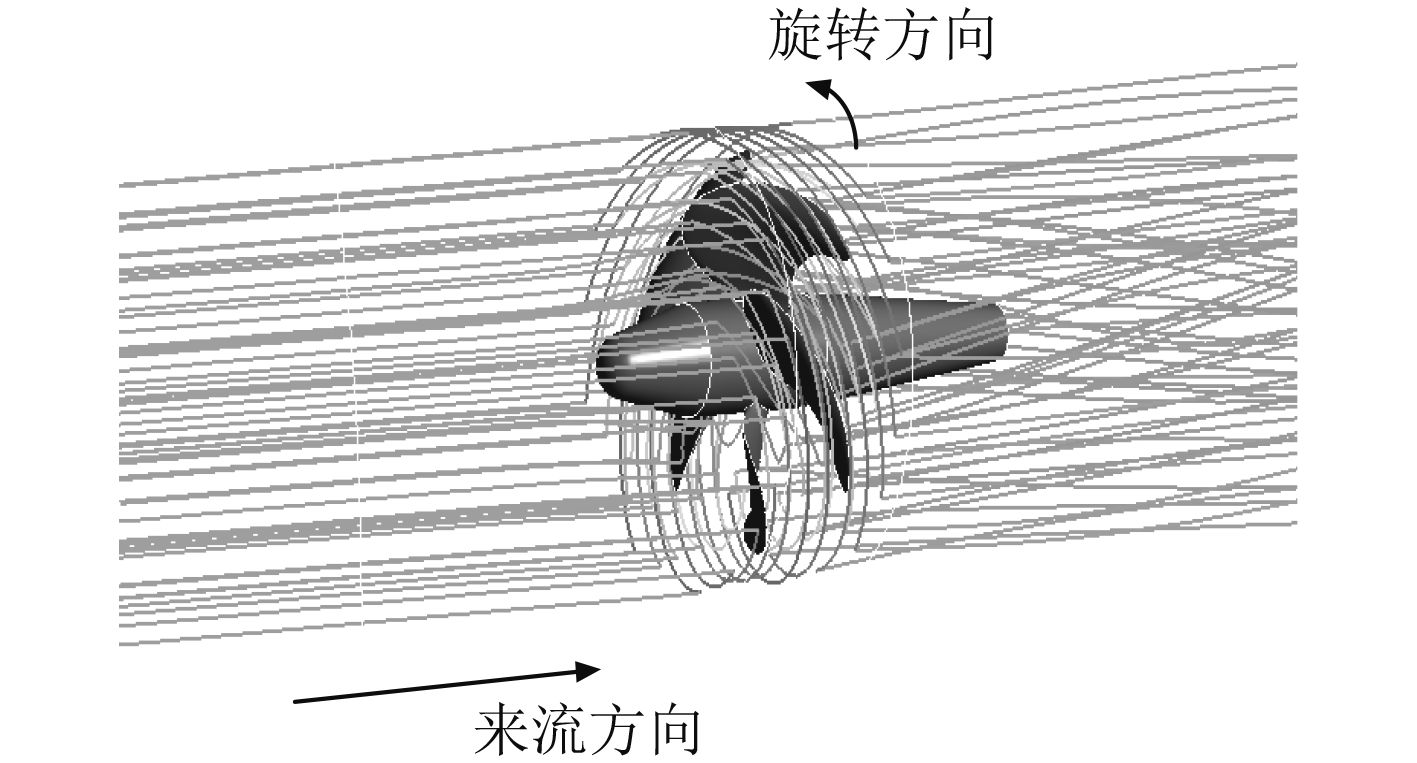图 5 调距桨前后流场流线分布 Fig. 5 Streamline distribution of CPP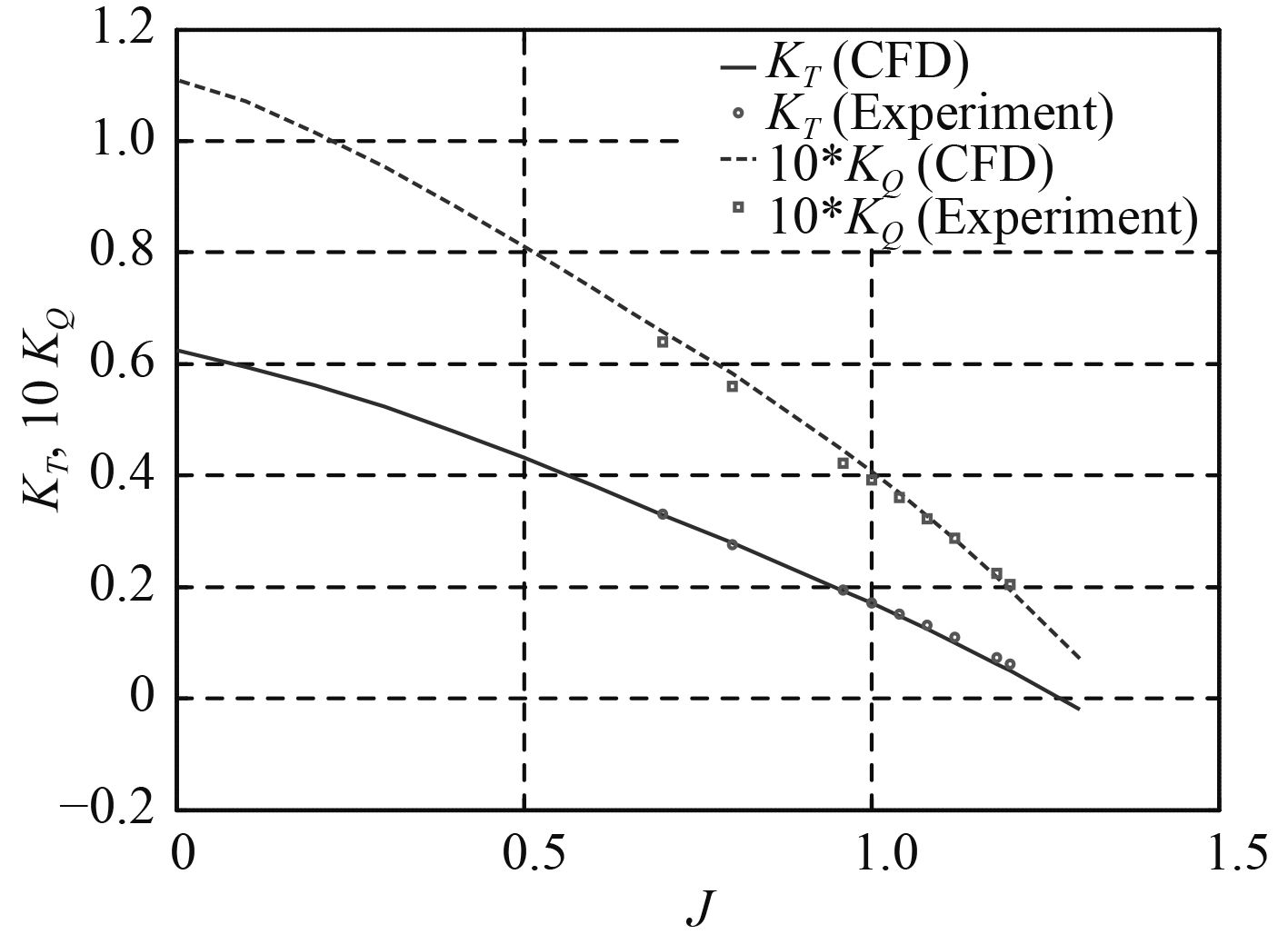图 6 调距桨敞水特性CFD计算结果校验 Fig. 6 CFD result verification of CPP performance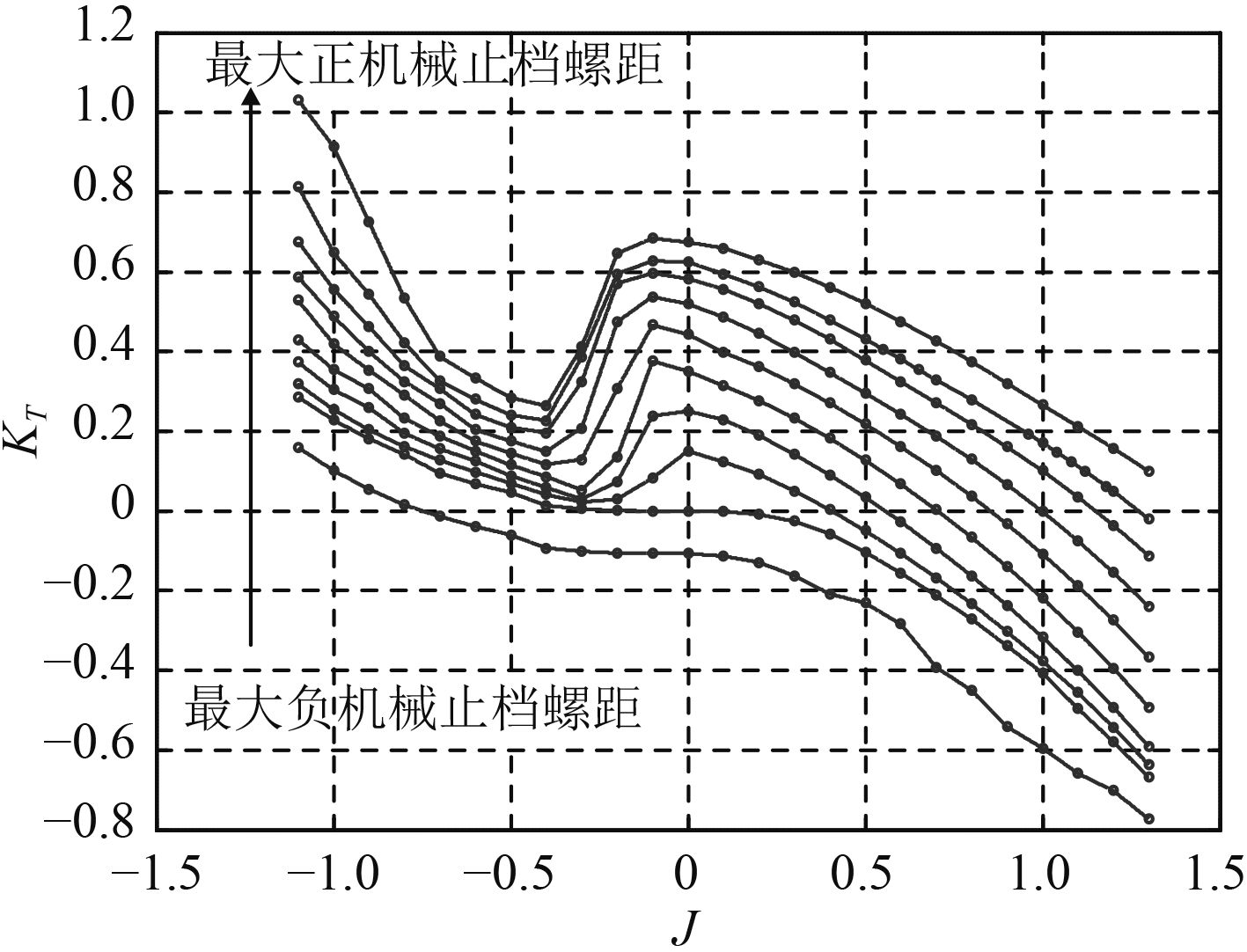图 7 调距桨推力系数CFD计算结果 Fig. 7 CFD result of KT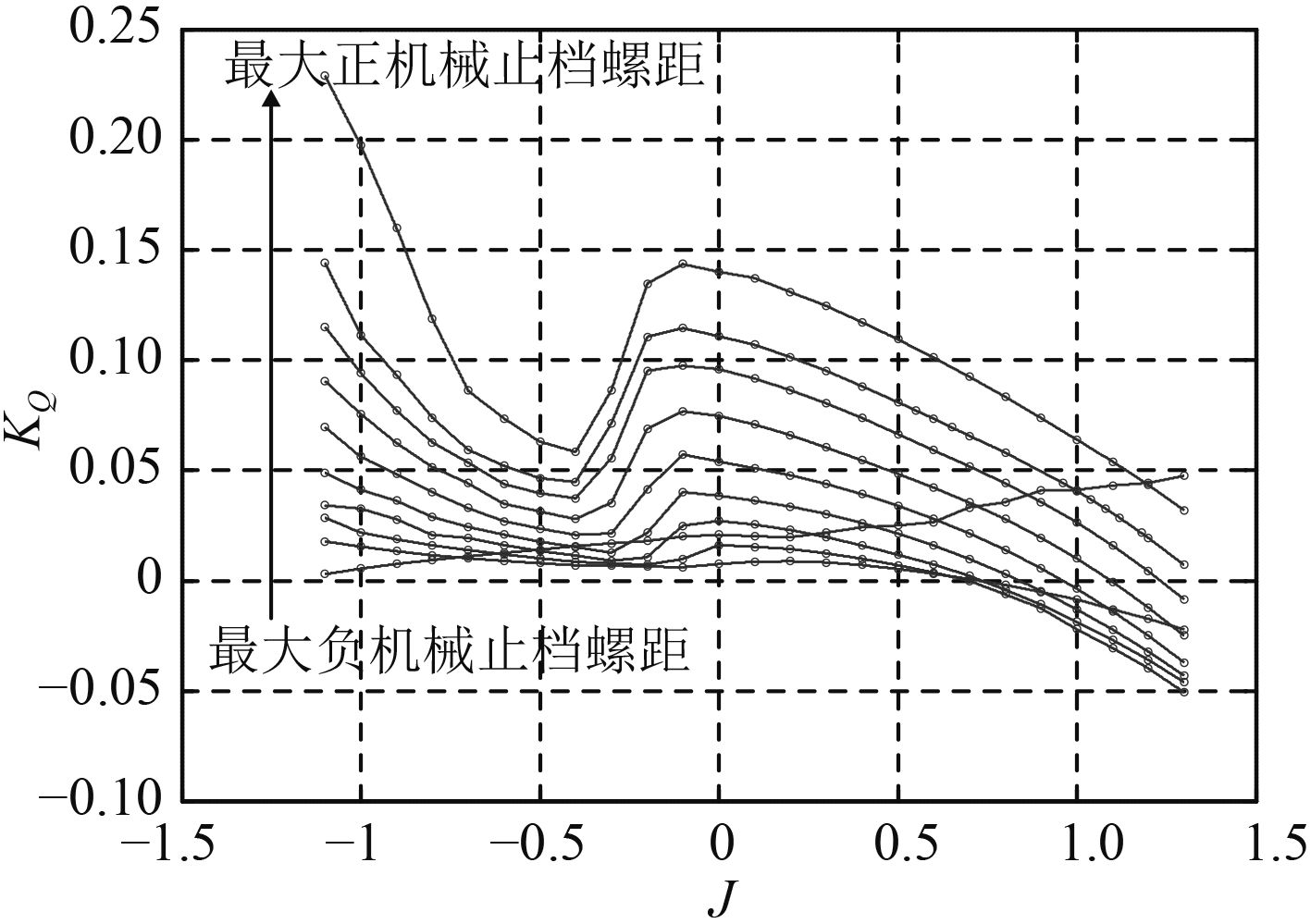图 8 调距桨力矩系数CFD计算结果 Fig. 8 CFD result of KQ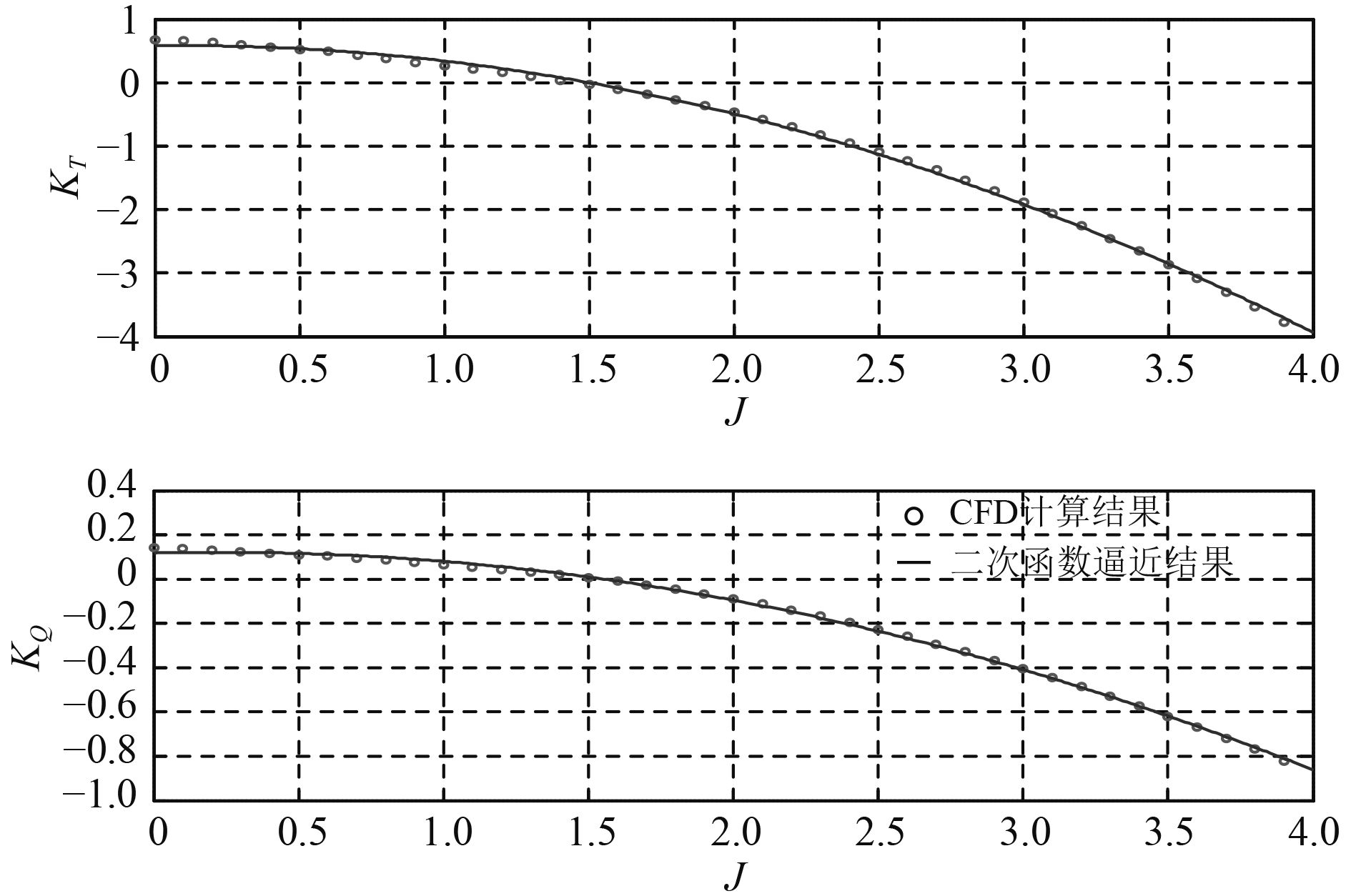图 9 大进速系数时的CFD计算结果与二次曲线拟合结果比较 Fig. 9 Compare between CFD and conic fit result under big advance ratio

2 CODOG推进系统仿真模型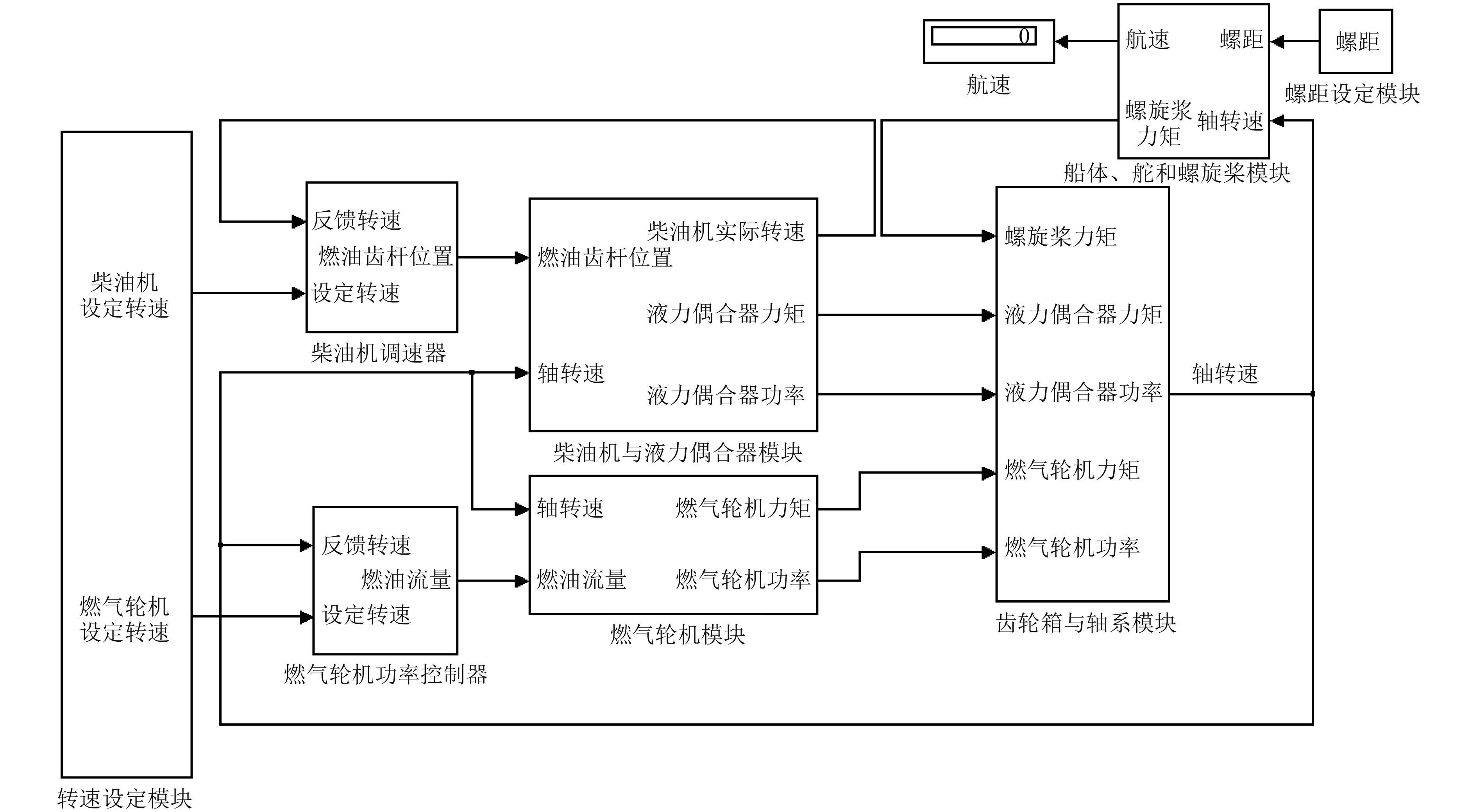图 10 CODOG推进系统仿真模型 Fig. 10 Simulation model of CODOG propulsion system
3 单桨工况仿真与分析

 ${T_p} - R - {T_R} = 0\text{。}$ (3)

 ${Q_R} - {Q_f} = 0\text{。}$ (4)

 $\left\{ \begin{array}{l}{K_T}\rho {n^2}{D^4} - R(V_s) - {K_{TR}}\rho {n_R}^2{D^4} = 0\text{，}\\{K_{QR}}\rho {n_R}^2{D^5} - {Q_f}({n_R}) = 0\text{。}\end{array} \right.$ (5)

3.1 主机工作在额定工况时的单桨工况仿真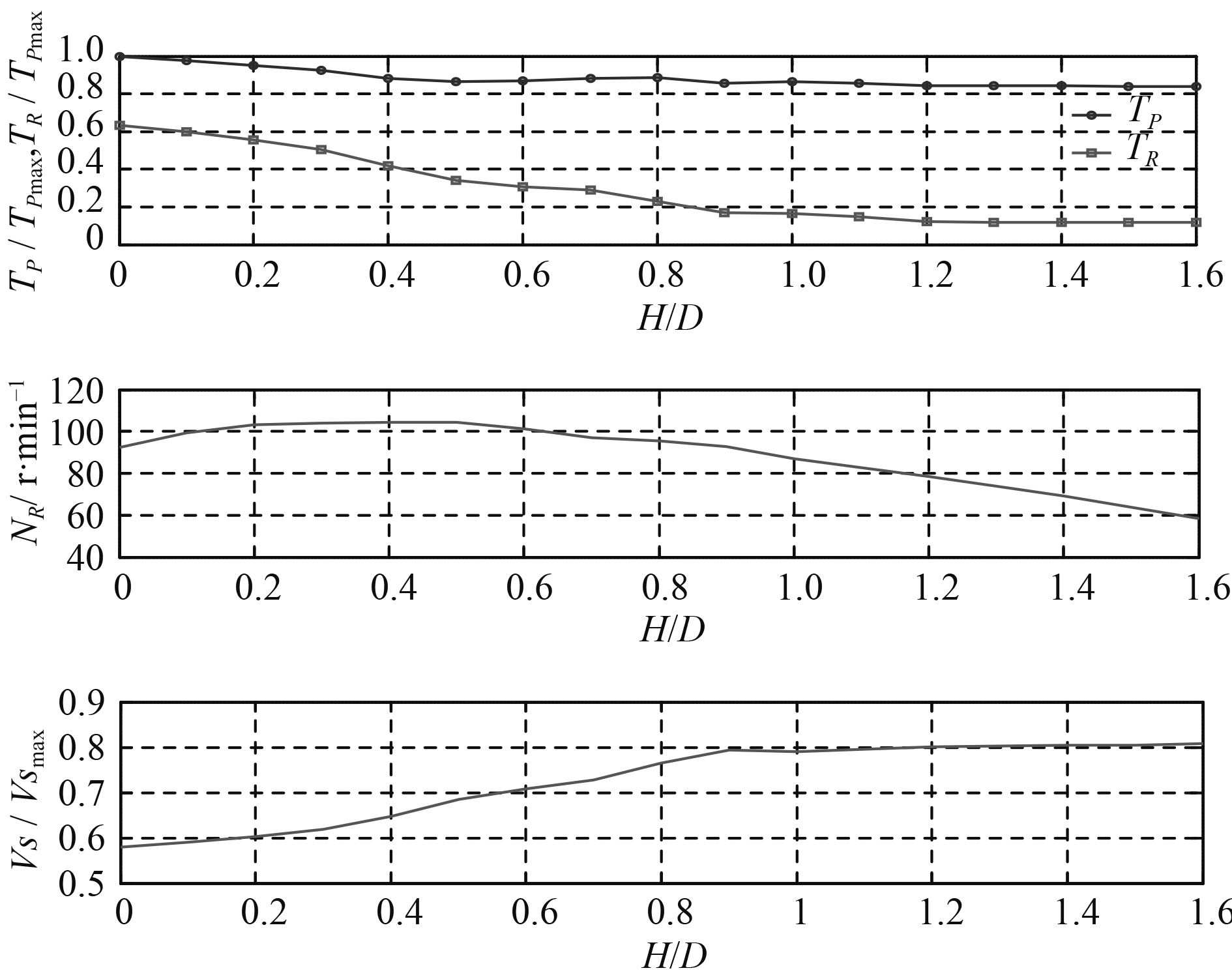图 11 柴油机单桨工况稳态特性仿真结果 Fig. 11 Steady-state characteristics simulation result of single diesel working condition

3.2 拖桨工况影响因素分析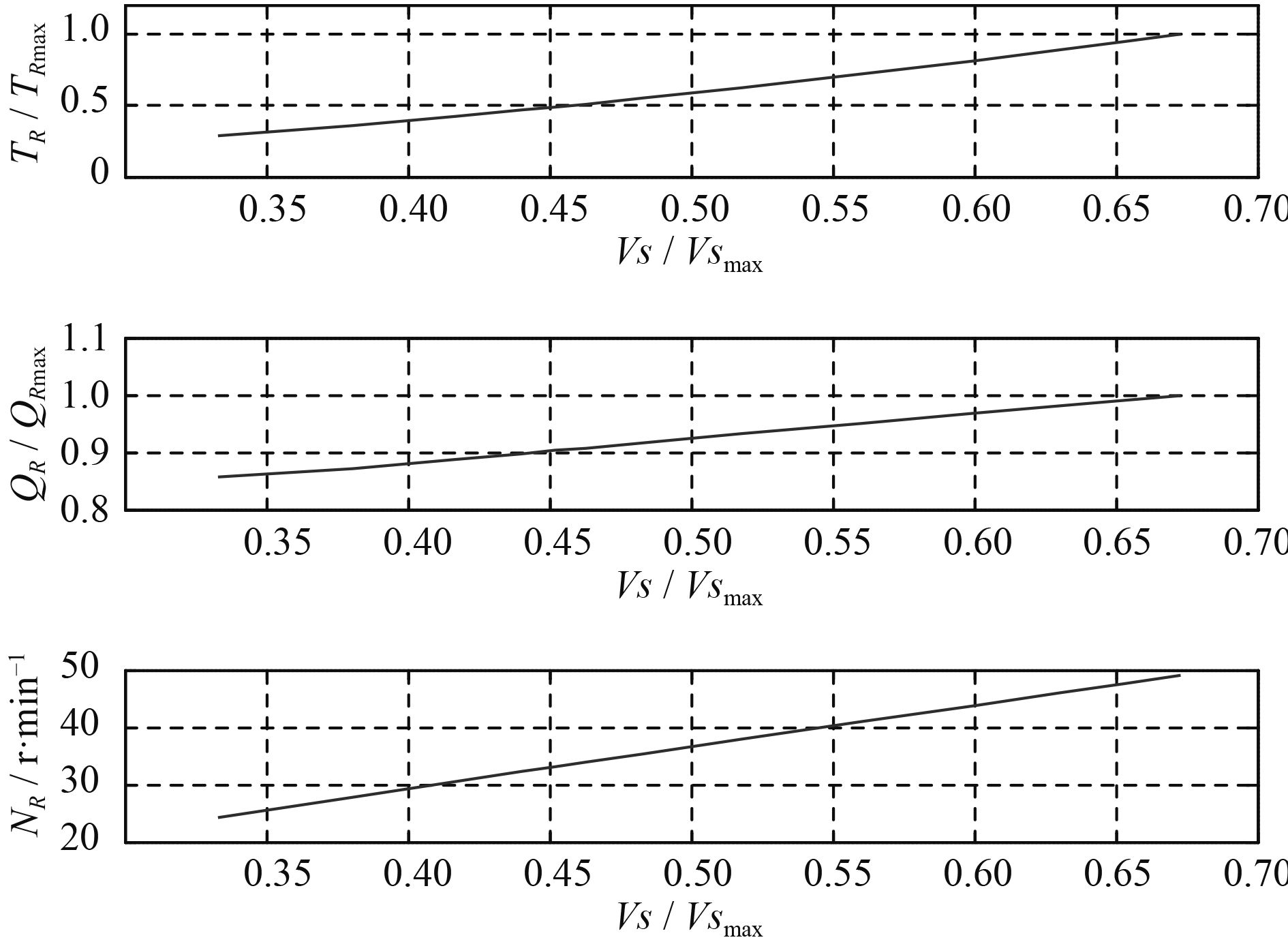图 12 拖桨阻力、力矩与转速随航速的变化关系 Fig. 12 Relationship between propeller’s dragged resistance, torque, rev and ship speed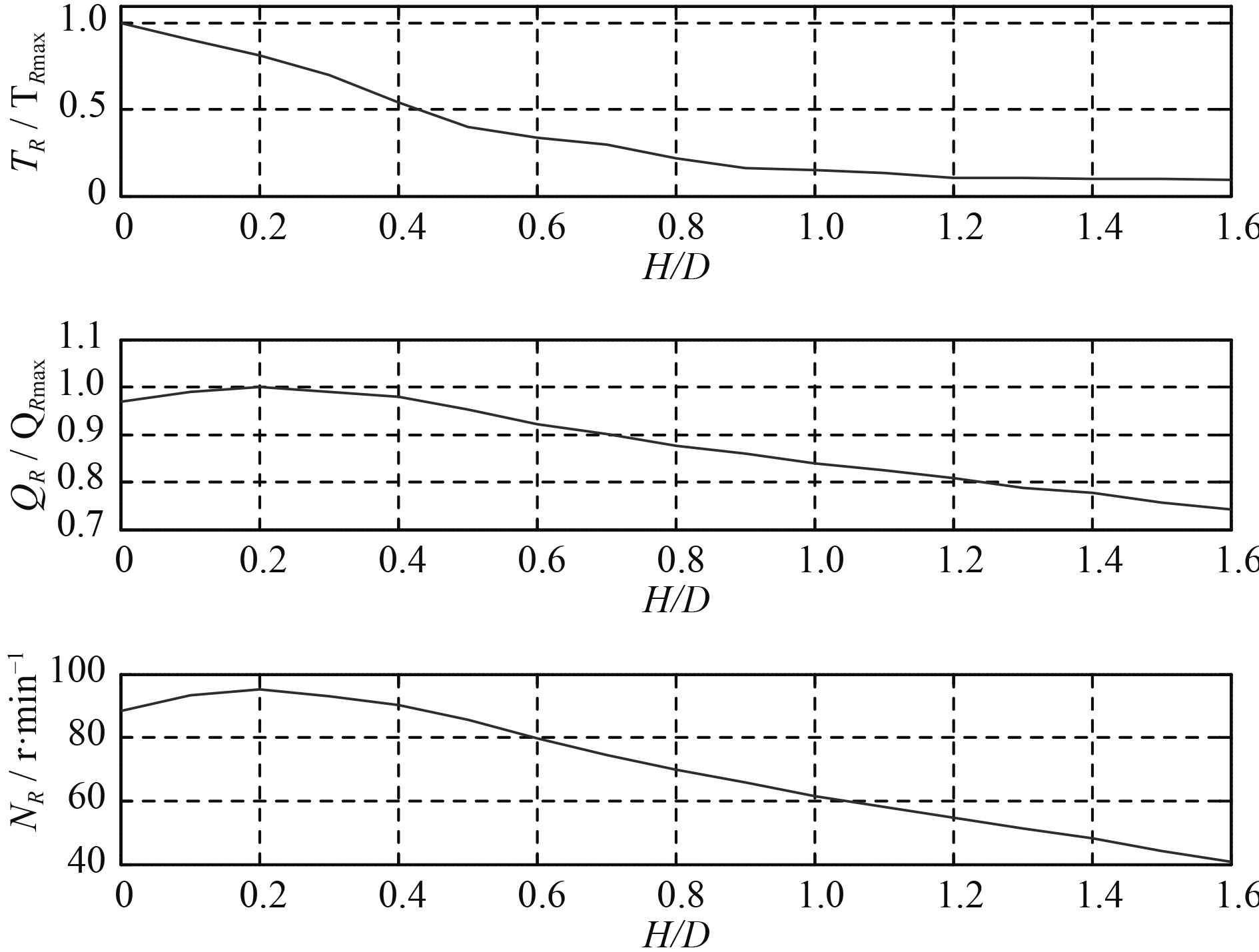图 13 拖桨阻力、力矩与转速随拖桨螺距的变化关系 Fig. 13 Relationship between propeller’s dragged resistance, torque, rev and pitch

可见航速VS和转桨螺距H/DR对拖桨工况的影响呈现明显的规律性。由于单桨工况下，TRQRnR直接或间接由方程组（5）确定，因此，对于工况1仿真得到的TRQRnRVS的变化规律，当H/DR变化时，拖桨工况的相关参数仍会有相同的规律；同理，对于工况2仿真得到的TRQRnRH/DR的变化规律，当VS变化时，拖桨工况的相关参数也有相同的规律。因此，当VSH/DR确定后，TRQRnR也随之确定。

4 结　语

1）单桨工况下，当不工作桨自由拖转时，将其螺距设定为最大值时有最小的拖桨阻力和拖桨力矩，船舶的快速性最好。

2）影响不工作桨水动力特性的因素有航速和不工作桨螺距2个。航速越高，拖桨阻力、拖桨力矩以及拖桨转速越大；不工作桨螺距越大，拖桨阻力越小，拖桨力矩与拖桨转速先略微增加而后减小至最小值。

  王永生. 全柴联合动力装置稳、动态特性和工作制研究[D]. 武汉: 华中科技大学, 2005.  杨琼方, 王永生, 张志宏, 等. 大侧斜螺旋桨敞水性能的RANSEs模拟[J]. 湖南科技大学学报, 2008, 23(4): 66–70. http://www.wanfangdata.com.cn/details/detail.do?_type=perio&id=xtkyxyxb200804016  王永生, 丁江明. 液力偶合器通用外特性的数学建模[J]. 机械工程学报, 2005, 41(4): 225–228. http://www.wanfangdata.com.cn/details/detail.do?_type=perio&id=jxgcxb200504045  单铁兵. 四桨两舵船舶螺旋桨不同工况下水动力性能及船体操纵性研究[D]. 哈尔滨: 哈尔滨工程大学, 2008.  WANG Yong-sheng, DENNIS N A, ZHANG Yu-sheng. A practical approach of developing mathematical model for all speed governors[J]. Journal of Shanghai Jiaotong University, 2005, 2: 175–181. http://www.wanfangdata.com.cn/details/detail.do?_type=perio&id=shjtdxxb-e200502016  林缨. 联合动力装置控制策略及其仿真研究[D]. 上海: 上海交通大学, 2004.  张维竞. 舰船动力装置系统仿真[M]. 上海: 上海交通大学出版社, 2007.